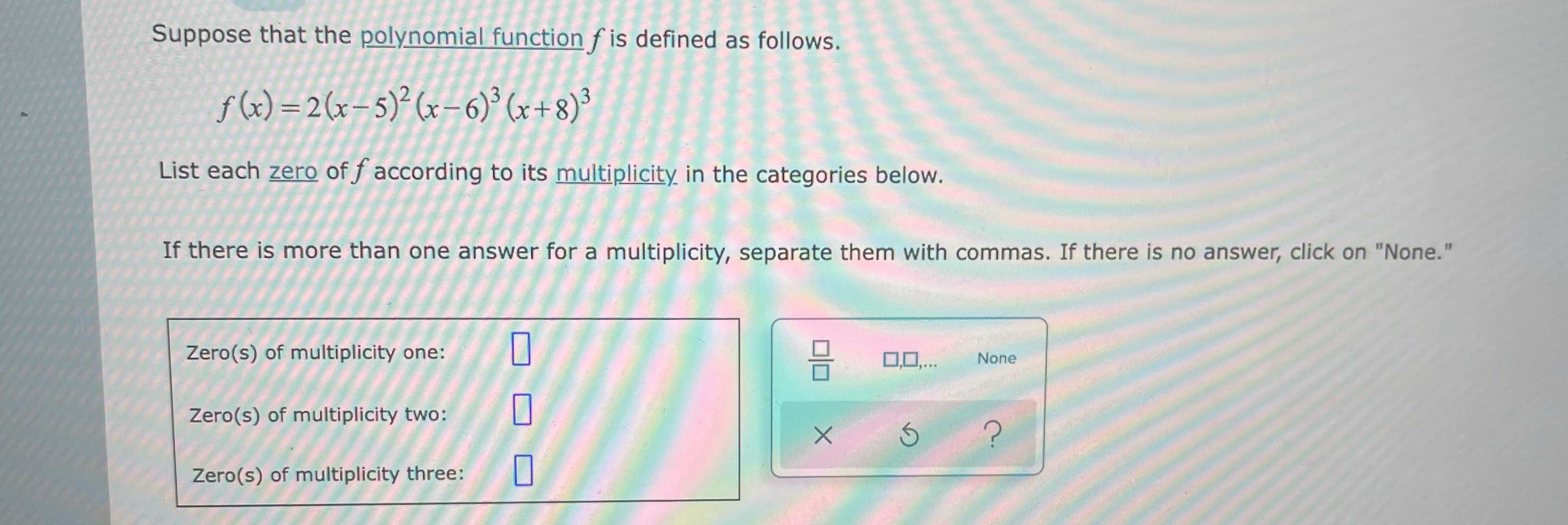### Still have math questions?

Algebra
QuestionSuppose that the polynomial function $$f$$ is defined as follows.

$$f ( x ) = 2 ( x - 5 ) ^ { 2 } ( x - 6 ) ^ { 3 } ( x + 8 ) ^ { 3 }$$

List each zero of $$f$$ according to its multiplicity in the categories below.

If there is more than one answer for a multiplicity, separate them with commas. If there is no answer, click on "None."

Zero(s) of multiplicity one:

Zero(s) of multiplicity two:

Zero(s) of multiplicity three: